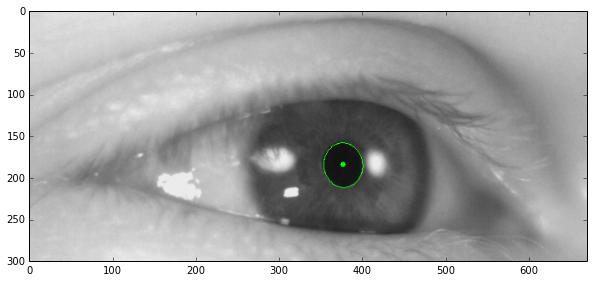# High Performance Eye-Tracking¶

## 001 - Pupil Detection¶

27.01.2014

Ultimately the goal is to robustly detect the presence and position of an eye in an image, as well as locate and identify as precisely and as quickly as possible all the individual features of the eye, pupil, iris, etc.

In :
from IPython.core.display import Image
Image(filename='001/eye-diagram.jpg')

Out: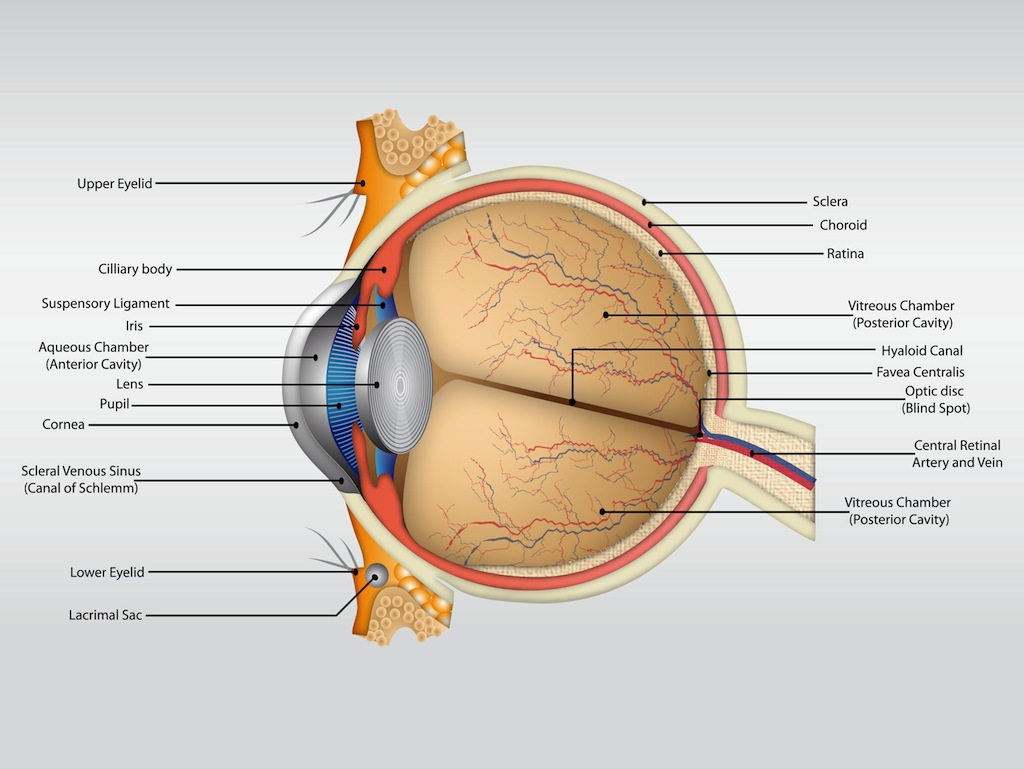In order to do this, I will start with detecting the pupil, because it offers good contrast ratio based on which it can be detected in most images. In this notebook I will look at different methods that can be used to detect the pupil and what their advantages and disadvantages are.

### Binary Thresholding¶

The idea behind binary thresholding is that it will force all pixels in an image that are darker than a certain threshold to black, and all the other pixels to white. Since the pupil is usually almost black, this should produce a circle of black surrounded by white, in the ideal case.

In :
%matplotlib inline
import cv2
import numpy as np
import matplotlib.pyplot as plt

gray = cv2.cvtColor(image, cv2.cv.CV_BGR2GRAY)

plt.figure(figsize=(10, 5))
plt.imshow(gray, cmap='gray')

Out:
<matplotlib.image.AxesImage at 0x10ddca890>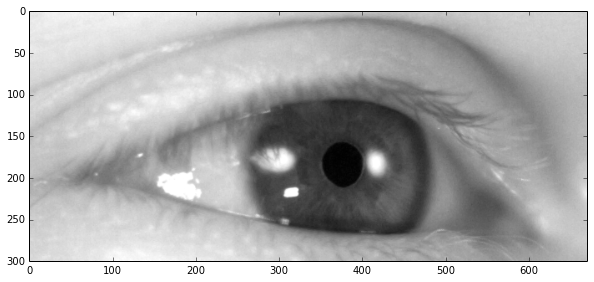Now that we've loaded the image, we can try thresholding it. It is worth noting that the image is now grayscale (0-255).

In :
retval, thresholded = cv2.threshold(gray, 30, 255, cv2.cv.CV_THRESH_BINARY)

plt.figure(figsize=(10, 5))
plt.imshow(thresholded, cmap='gray')

Out:
<matplotlib.image.AxesImage at 0x10dcb6990>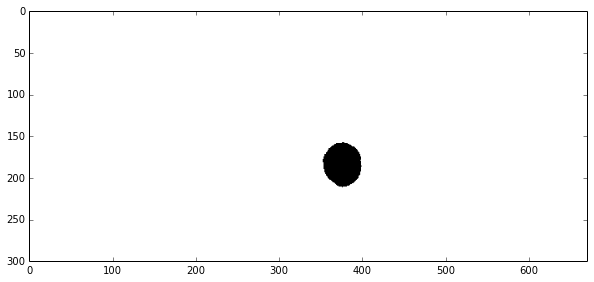Now this particular result is very good, it shows theprecise position of the pupil and nothing else! But what if the pupil was over the threshold of 30?

Now draw that pupil back into the original image

In :
kernel = cv2.getStructuringElement(cv2.MORPH_RECT, (10, 10))
closed = cv2.erode(cv2.dilate(thresholded, kernel, iterations=1), kernel, iterations=1)

plt.figure(figsize=(10, 5))
plt.imshow(closed, cmap='gray')

Out:
<matplotlib.image.AxesImage at 0x10dce6710>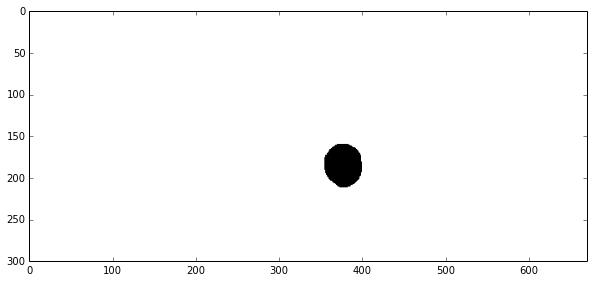In :
contours, hierarchy = cv2.findContours(closed, cv2.cv.CV_RETR_LIST, cv2.cv.CV_CHAIN_APPROX_NONE)

drawing = np.copy(image)

for contour in contours:
area = cv2.contourArea(contour)
bounding_box = cv2.boundingRect(contour)

extend = area / (bounding_box * bounding_box)

# reject the contours with big extend
if extend > 0.8:
continue

# calculate countour center and draw a dot there
m = cv2.moments(contour)
if m['m00'] != 0:
center = (int(m['m10'] / m['m00']), int(m['m01'] / m['m00']))
cv2.circle(drawing, center, 3, (0, 255, 0), -1)

# fit an ellipse around the contour and draw it into the image
ellipse = cv2.fitEllipse(contour)
cv2.ellipse(drawing, box=ellipse, color=(0, 255, 0))

plt.figure(figsize=(10, 5))
plt.imshow(drawing)

Out:
<matplotlib.image.AxesImage at 0x1102b8d50>